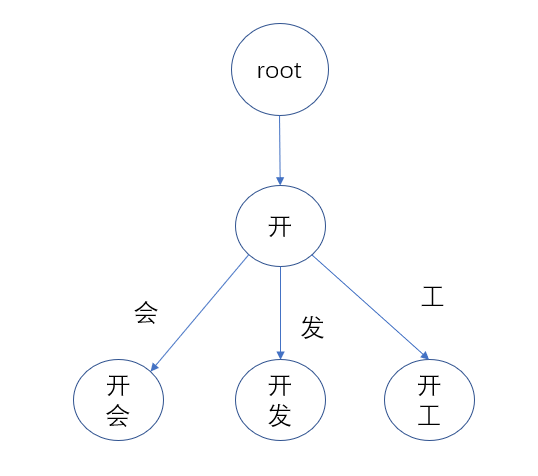7212字，阅读需时25分钟# 分词结构
class SegmentWord:
def __init__(self,word,wordAtt,index):
# 分词
self.word = word
# 分词词性
self.wordAtt = wordAtt
# 分词在文本中的位置
self.index = index# 节点类
class TrieNode(object):
def __init__(self):
# 字典的key为字符
# 字典的value为TrieNode
self.child = {}
# 标志位，为1表示单词结束
self.flag = None

Trie树类
# 树类
class Trie(object):
def __init__(self):
self.root = TrieNode()

# 添加单词
curNode = self.root
for word in words:
if curNode.child.get(word) is None:
nextNode = TrieNode()
curNode.child[word] = nextNode
curNode = curNode.child[word]
curNode.flag = 1

# 精准查找
def search_exact(self, words):
curNode = self.root
for word in words:
if curNode.child.get(word) is None:
return False
else:
print(word)
curNode = curNode.child[word]
if curNode.flag == 1:
return True

# 模糊查找
def search_fuzzy(self, sentence):
curNode = self.root
for word in sentence:
if curNode.child.get(word) is None:
return False
else:
print(word)
if curNode.child[word].flag == 1:
return True
else:
curNode = curNode.child[word]
return True
# 输出单词
def print_words(self):
rootnode = self.root
for key in rootnode.child.keys():
print(key)
self.print_sub_words(rootnode.child[key])
# 输出子节点字符
def print_sub_words(self,node):
for key in node.child.keys():
print(key)
self.print_sub_words(node.child[key])

# 读取词典文件
filename = "sample.txt"
try:
#使用r模式打开文本文件
fp = open(filename,"r",encoding='utf-8')
print("%s 文件打开成功" % filename)
done = False
#使用while循环读取文本文件所有行数
while not done:
#按行读取文件内容
if(aline != ""):
# 过滤换行符
words = aline.replace('\r','').replace('\n','').replace('\t','')
# 添加单词到Trie树
else:
done = True
#关闭文件对象
fp.close()
except IOError:
print("文件打开失败，%s文件不存在" % filename)

sample.txt和Python程序在同一个目录，sample.txt存储的单词如下：

def  forward_max_matching(sentence,trie):
words = []
# 句子的字符数
sentence_len = len(sentence)
if sentence_len <= 0:
return
# 起始索引
start = 0
# 终止索引
end = 1
temp = ""
while True:
# 提取分词
word = sentence[start:end]
# 若当前分词匹配成功，添加字符继续匹配
if trie.search_exact(word):
end += 1
temp = word
if end >= sentence_len:
words.append(temp)
break
else:
# 若temp不为空，添加分词到words
if len(temp) > 0:
words.append(temp)
start += len(temp)
temp = ""
# 终止索引加1
end += 1
if end > sentence_len:
break
return  words

Python分词工具很多，包括盘古分词、Yaha分词、Jieba分词、清华THULAC等，这些分词程序都是开源软件，在许可协议下，可以免费使用这些分词程序。